## Recommended Posts

Albert Einstein did introduce E=mc^2 historically. I have seen numerous proofs for this relativisticly or by calculus from Newtons laws. However for some time ago I found a site that described that a scientist did prove E=mc^2 from an energetic perspective by adding together all energy available from different energy types. I can't find this site online now unfortunately. So I wonder if anyone know the name of this proof for E=mc^2 by adding together different energy contributions. I don't want a derivation I simply want a site that describes this theory or the name of the theory if it has one.

I believe the proof was introduced a bit later then Einsteins proof perhaps later then 1930. I cant remember if it was semiemperical or not.

Edited by Tor Fredrik

##### Share on other sites

People have done calculations of e.g. the self-energy of charged particles, i.e. assuming you are assembling the particle out of some nebulous stuff that has an average charge density, but these are incorrect. You get, for example, the classical electron radius from equating the self-energy to the mass energy, but experiment shows that the electron doesn't have such a radius and is in fact consistent with being a point particle.

I'm not sure what other energy types you might be talking about, where E=mc^2 isn't already being applied.

##### Share on other sites

It does not have to be a new theorem where E=mc^2 has not been applied. But a quantification of why E=mc^2 is legit. I am pretty sure I read something about it. Could you write a list over all proofs of E=mc^2 that you know? Semi emperical and not emperical. Would be great.

##### Share on other sites
3 minutes ago, Tor Fredrik said:

It does not have to be a new theorem where E=mc^2 has not been applied. But a quantification of why E=mc^2 is legit. I am pretty sure I read something about it. Could you write a list over all proofs of E=mc^2 that you know? Semi emperical and not emperical. Would be great.

A quantification of why the equation is legit would include any experiment that confirms isotopic masses and decay Q-values. There are hundreds of examples.

##### Share on other sites

I have tried to work on a derivation. Here is what I obtained.But my problem is how to get from an arbitrary velocity v to the speed of light.

##### Share on other sites
11 hours ago, Tor Fredrik said:

I have tried to work on a derivation. Here is what I obtained.

But my problem is how to get from an arbitrary velocity v to the speed of light.

The problem is that the velocity of the particle in this scenario is unrelated to c. It's not a Newtonian issue, so a Newtonian derivation is probably not going to get you there.

##### Share on other sites
On 11/18/2019 at 11:33 AM, Tor Fredrik said:

However for some time ago I found a site that described that a scientist did prove E=mc^2 from an energetic perspective by adding together all energy available from different energy types.

Prior annihilation of electron with positron energy of both particles is:

E= 2 mec2

After annihilation there are two gamma photons with energies:

E = 2 h fc

2 mec2 = 2 h fc

Reverse process is called pair-production.

Compton scattering relies heavily on it as well.

##### Share on other sites
59 minutes ago, Sensei said:

Prior annihilation of electron with positron energy of both particles is:

E= 2 mec2

After annihilation there are two gamma photons with energies:

E = 2 h fc

2 mec2 = 2 h fc

Reverse process is called pair-production.

That's experimental confirmation, rather than a derivation. The OP's previous post suggests that a derivation is being sought.

##### Share on other sites
18 minutes ago, swansont said:

That's experimental confirmation, rather than a derivation. The OP's previous post suggests that a derivation is being sought.

In the first post I read:

On 11/18/2019 at 11:33 AM, Tor Fredrik said:

I don't want a derivation

##### Share on other sites

Thanks for all the response. I have moved on and looked at a different derivation that uses E=hf.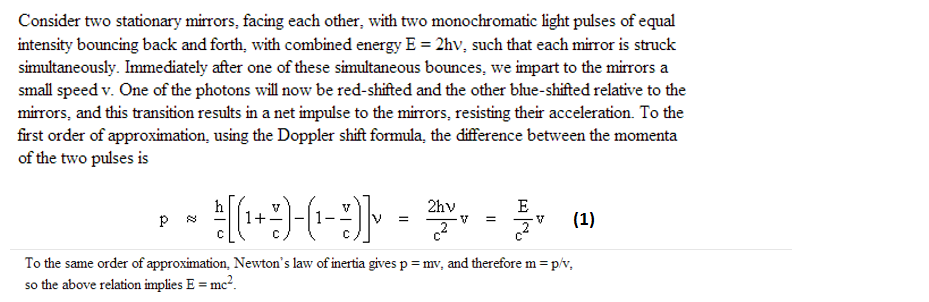The formula that I have derived for the doppler shift formula is for this casegives this corrected wavelength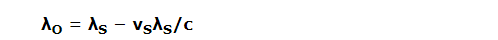And this casegives this observed wavelength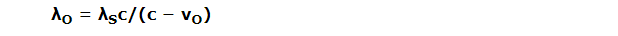But is this applicable to the momentum formula in (1). And how do they use plancks constant h in the momentum approxiamtion to the left of (1). Can someone derive this momentum approximation if possible?

Edited by Tor Fredrik

##### Share on other sites

I have wanted to edit the post above. But since I have not managed to edit I will write an update. Further derivation for the frequency is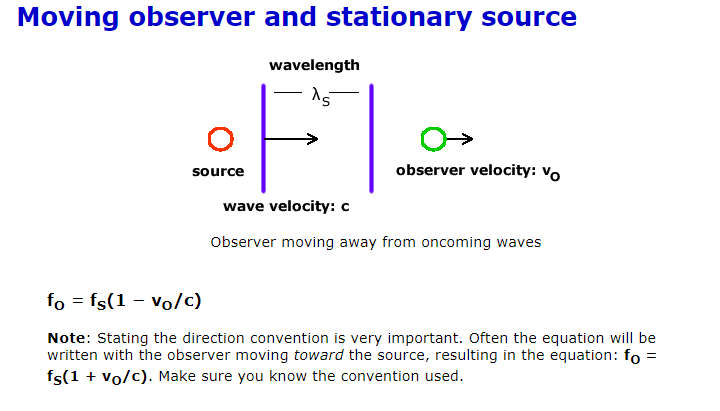'so if a photon between the two mirrors are moving then we would get for the mirror that moves away from the photon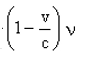As for the other mirror I am not sure. It does move away from the photon as well but (1) uses toward the source. And why are there two photons needed for this derivation? In short I am a bit lost over the energy translation here between the two mirrors and the photons.  I am aware that they use p=E/c introduced by Maxwell.

Edited by Tor Fredrik

## Create an account

Register a new account